# fasstr Users Guide

fasstr, the Flow Analysis Summary Statistics Tool for R, is a set of R functions to tidy, summarize, analyze, trend, and visualize streamflow data. This package summarizes continuous daily mean streamflow data into various daily, monthly, annual, and long-term statistics, completes trending and frequency analyses, with outputs in both table and plot formats.

This vignette documents the usage of the many functions and arguments provided in fasstr. This vignette is a high-level adjunct to the details found in the various function documentations (see help(package = "fasstr") for documentation). You’ll learn how to install the package and a HYDAT database, input data into fasstr functions, add relevant columns and rows to daily data, screen data for outliers and missing dates, calculate and visualize various summary statistics, trend annual flows, and complete volume frequency analyses.

A quick reference PDF cheat sheet is also available for fasstr usage of functions and arguments. It can be downloaded here.

This guide contains the following sections to help understand the usage of the fasstr functions and arguments:

1. Getting Started
2. Flow Data Inputs
3. Function Outputs
4. Data Tidying (fill_* and add_* functions)
5. Data Screening (screen_* functions)
6. Calculating Statistics (calc_* functions)
7. Analyses (compute_* functions)
8. Customizing Functions - Data filtering and options
9. Writing Tables and Plots (write_* functions)

## 1. Getting Started

You can install fasstr directly from CRAN:

install.packages("fasstr")

To install the development version from GitHub, use the remotes package then the fasstr package:

if(!requireNamespace("remotes")) install.packages("remotes")
remotes::install_github("bcgov/fasstr")

Several other packages will be installed with fasstr. These include tidyhydat for downloading Water Survey of Canada hydrometric data, zyp for trending, ggplot2 for creating plots, and tidyr and dplyr for data wrangling and summarizing, amongst others.

To call fasstr functions you can either load the package using the library() function or access a specific function using a double-colon (e.g. fasstr::calc_daily_stats()). fasstr exports the pipe, %>%, so it can be used for tidy workflows.

library(fasstr)

To use the station_number argument of the fasstr functions, you will need to download a Water Survey of Canada HYDAT database to your computer using the following tidyhydat function. The function will save the database on your computer and know where to find it each time you open R or RStudio. Due to the size of the database, it will take several minutes to download.

tidyhydat::download_hydat()

As HYDAT is updated frequently you may want to periodically update it yourself using the function above. You can check the local version using the following code:

tidyhydat::hy_version()

## 2. Flow Data Inputs

All functions in fasstr require a daily mean streamflow data set from one or more hydrometric stations. Long-term and continuous data sets are preferred for most analyses, but seasonal and partial data can be used. Note that if partial data sets are used, NA‘s may be produced for certain statistics. Please see the ’Handling Missing Dates’ section in Section 8 for more information. Data is provided to each function using one of the following arguments:

• data, as a data frame of daily flow values, or
• station_number, as a list of Water Survey of Canada HYDAT station numbers.

### data (and dates, values, and groups)

Using the data option, a data frame of daily data containing columns of dates (YYYY-MM-DD in date format), values (mean daily discharge in cubic metres per second in numeric format), and, optionally, grouping identifiers (character string of station names or numbers) is called. By default, the functions will look for columns identified as ‘Date’, ‘Value’, and ‘STATION_NUMBER’, respectively, to be compatible with the HYDAT default columns. However, columns of different names can be identified using the dates, values, groups column arguments (ex. values = Yield_mm). The values of these arguments are not required to be surrounded by quotes; both "Date" and Date will provide the appropriate column called “Date”. An example where groupings other than station numbers could be used include certain time periods of a study for a single station (before, during, and after watershed experiment treatments or before and after the construction of a dam, appropriately identified in a column). The following is an example of an appropriate data frame with default column names (STATION_NUMBER not required):

  STATION_NUMBER       Date Value
1        08NM116 1949-04-01  1.13
2        08NM116 1949-04-02  1.53
3        08NM116 1949-04-03  2.07
4        08NM116 1949-04-04  2.07
5        08NM116 1949-04-05  2.21
6        08NM116 1949-04-06  2.21

The following is an example fasstr function arguments if your daily data data frame has the default columns names (no need to list them):

calc_longterm_daily_stats(data = flow_data)

The following is an example if your daily data data frame has non-default columns names of “Stations”, “Dates”, and “Flows”:

calc_longterm_daily_stats(data = flow_data,
dates = Dates,
values = Flows,
groups = Stations)

The data argument is listed first in the list of arguments for each function, so flow data frames can be passed onto fasstr functions using the pipe operator, %>%, without listing the data frame in a tidy workflow.

### station_number

Alternatively, you can directly extract flow data directly from a HYDAT database by listing station numbers in the station_number argument while leaving the data arguments blank. Data frames from HYDAT also include ‘Parameter’ and ‘Symbol’ columns. The following is an example of listing stations:

calc_longterm_daily_stats(station_number = "08NM116")
calc_longterm_daily_stats(station_number = c("08NM116", "08NM242"))

This package allows for multiple stations (or other groupings) to be analyzed in many of the functions; provided they are identified using the groups column argument (defaults to STATION_NUMBER). If named grouping column doesn’t exist or is improperly named then all values listed in the values column will be summarized.

## 3. Function Types and Outputs

fasstr provides various functions to help in streamflow analyses. They can be generally categorized into the following groups (with more details in the sections below):

• data tidying (to prepare data for analyses; add_* and fill_* functions),
• data screening (to look for outliers and missing data; screen_* functions),
• calculating summary statistics (long-term, annual, monthly and daily statistics; calc_*functions),
• computing analyses (volume frequency analyses and trending; compute_* functions),
• visualizing data (plotting the various statistics; plot_* functions), and
• writing data (to save your data and plots; write_* functions)

### Tibble Data Frames

Functions that produce tables create them as tibble data frames. To facilitate the writing of the fasstr tibbles to a directory as .csv, .xls, or .xlsx files with some functionality of rounding digits, the write_results() function can be used (see section 9 for more information).

### ggplot2 Plots

Functions that produce plots create them as lists of ggplot2 objects. The use of ggplot2 plots allows for further customization of plots for the user (axis titles, colours, etc.). All plotting functions produce lists to be consistent with table naming conventions of fasstr, allow multiple plots to be created with one function, and to easily allow the saving of multiple plots to a directory. To assist with the saving of lists of plots, a provided function called write_plots() will directly save the list of plots within a directory or single PDF document, with the fasstr plot objects names (see section 9 for more information). Individual plots can be subsetted from their lists using either the dollar sign, $(e.g. one_plot <- plots$plotname), or double square brackets, [ ] (e.g. one_plot <- plots[[plotname]] or one_plot <- plots[]).

Some functions produce both tibbles and plots as lists and can be subsequently subsetted as desired.

## 4. Data Tidying Functions

There are several functions that are used to prepare your flow data set for your own analysis. These functions begin with add_ or fill_ and add columns or rows, respectively, to your flow data frame. These functions include:

• fill_missing_dates() - fills in missing dates or dates with no flow values with NA
• add_date_variables() - add year, month, and day of year variables (and water years if selected)
• add_seasons() - add a column of seasons
• add_rolling_means() - add rolling n-day averages (e.g. 7-day rolling average)
• add_basin_area() - add a basin area column to daily flows
• add_daily_volume() - add daily volumetric flows (in cubic metres)
• add_daily_yield() - add daily water yields (in millimetres)
• add_cumulative_volume() - add daily cumulative volumetric flows on an annual basis (in cubic metres)
• add_cumulative_yield() - add daily cumulative water yields on an annual basis (in millimetres)

The functions are set up to easily incorporate the use of the pipe operator:

fill_missing_dates(station_number = "08HA011") %>%
add_rolling_means(roll_days = 7)
  STATION_NUMBER       Date Parameter Value Symbol CalendarYear Month MonthName
1        08HA011 1960-01-01      Flow  62.9      E         1960     1       Jan
2        08HA011 1960-01-02      Flow  58.0      E         1960     1       Jan
3        08HA011 1960-01-03      Flow  54.9      E         1960     1       Jan
4        08HA011 1960-01-04      Flow  51.3      E         1960     1       Jan
5        08HA011 1960-01-05      Flow  47.3   <NA>         1960     1       Jan
6        08HA011 1960-01-06      Flow  46.7   <NA>         1960     1       Jan
WaterYear DayofYear Q7Day
1      1960         1    NA
2      1960         2    NA
3      1960         3    NA
4      1960         4    NA
5      1960         5    NA
6      1960         6    NA

### Filling missing dates

To ensure that analyses do not skip over dates, the fill_missing_dates() function looks for gaps in dates and adds the dates and fills in the flow values with NA. It does not do any gap filling (linear or correlations, for example), it assigns missing flow values with NA. It also fills dates to create complete start and end years. For example, if data starts in April, all flow values starting from January will be filled with NA. The timing of the year depends on the water_year_start argument. When water_year_start is left blank, it will fill to complete calendar years (Jan-Dec). If water_year_start is set to another month (numeric) then it will fill to complete water years of the desired year.

Run and compare the following lines to see how missing dates are filled:

# Very gappy (early years):
tidyhydat::hy_daily_flows(station_number = "08NM116")

# Gap filled with NA's
tidyhydat::hy_daily_flows(station_number = "08NM116") %>%
fill_missing_dates()

It is ideal to fill missing dates before using other add_* functions so dates added are not missing the other new date values.

### Adding date variables and seasons

The add_date_variables() function adds useful dates columns for summarizing data. The function defaults include ‘CalendarYear’, ‘Month’ (numeric), ‘MonthName’ (month abbreviation; e.g. Jan), ‘WaterYear’ (year based on selected water_year_start), and ‘DayofYear’ (the day of year based on selected water_year_start from 1-365). The month of the start of the water year is chosen using the water_year_start argument, which defaults to “1” for January.

Run and compare the following lines to see how the date columns are added:

# Just calendar year info

# If water years are required starting August (use month number)
water_year_start = 8)

The add_seasons() function adds a column of seasons identifiers called “Season”. The length of seasons, in months, is provided using the seasons_length argument. As seasons are grouped by months the length of the seasons must be divisible into 12 with season lengths of 1, 2, 3, 4, 6, or 12 months. The start of the first season coincides with the start month of each year; ‘Jan-Jun’ for 6-month seasons starting with calendar years or ‘Dec-Feb’ for 3-month seasons starting with water year starting in December. Run and compare the following lines to see how seasons columns are added:

#  2 seasons starting January
seasons_length = 6)

#  4 seasons starting October
water_year_start = 10,
seasons_length = 3)

#  4 Seasons starting December
water_year_start = 12,
seasons_length = 3)

Adding rolling means (running means or averages) of daily data, can be done using the add_rolling_means() functions. Based on the selected “n” rolling days using the roll_days argument, a column for each “n” will be added. One rolling mean column can be added by listing one number (e.g. roll_days = 7) or multiple columns can be added by listing each one (e.g. roll_days = c(3,7,30)). Each column will be named “Q’n’Day” where n is the number (e.g. Q7Day or Q30Day).

Where the alignment of the rolling mean is compared to the date is important to know when analyzing data. The alignment, using the roll_align argument, determine the date at which the rolling means occur.

• roll_align = "right" - the date will have the mean of that date’s flow value and the previous n-1 days
• roll_align = "left" - the date will have the mean of that date’s flow value and the next n-1 days
• roll_align = "center"
• odd numbered roll_days - date will have the mean of that date’s flow value and half of n-1 days before and half of n-1 days after
• even numbered roll_days - date will have the mean of that date’s flow and half of n days after, and the remaining before ((n/2)-1 days before the date) (i.e. the first of the middle two dates)

        Date Value Q5Day_left Q5Day_center Q5Day_right
1 1960-01-01  62.9      54.88           NA          NA
2 1960-01-02  58.0      51.64           NA          NA
3 1960-01-03  54.9      48.82        54.88          NA
4 1960-01-04  51.3      46.22        51.64          NA
5 1960-01-05  47.3      44.12        48.82       54.88
6 1960-01-06  46.7      42.36        46.22       51.64

Even roll_days example:

        Date Value Q6Day_left Q6Day_center Q6Day_right
1 1960-01-01  62.9   53.51667           NA          NA
2 1960-01-02  58.0   50.35000           NA          NA
3 1960-01-03  54.9   47.66667     53.51667          NA
4 1960-01-04  51.3   45.31667     50.35000          NA
5 1960-01-05  47.3   43.18333     47.66667          NA
6 1960-01-06  46.7   41.53333     45.31667    53.51667

To add a column of basin areas, for viewing or analyzing, the add_basin_area() function can be used. The basin area will be extracted from HYDAT, if available, under two conditions where the basin_area argument can be left blank:

• if the station_number argument is used
• if your data data frame has a grouping column consisting of HYDAT station numbers

If you would like to apply your own basin area size(s) or override the HYDAT areas, you use the basin_area argument in the following ways:

• for a single station or applying to all stations, list a single number (i.e. basin_area = 800)
• for different areas for multiple stations, you list each basin area for each station (i.e. basin_area = c("08NM116" = 800, "08NM242" = 4))

Run and compare the following lines to see how basin area columns are added:

# Using the station_number argument or data frame as HYDAT groupings

# Using the basin_area argument
basin_area = 800)

# Using the basin_area argument with multiple stations
basin_area = c("08NM116" = 800, "08NM242" = 4))

### Adding daily volumetric discharge or water yields

Converting daily mean discharge into other units can be useful for different analyses. Columns of total daily discharge converted from daily mean into volumetric flows, named “Volume_m3” in cubic metres per second, or area-based water yields, named “Yield_mm” in millimetres, can be used using the add_daily_volume() and add_daily_yield() functions, respectively. Volumetric gives the total volume per day, and the water yield gives the total water depth, provided an upstream drainage basin area is provided. Basin area can be provided using the basin_area argument, or if there is a groups column of HYDAT station numbers in your data then it will automatically be extracted from HYDAT, if available. (see adding basin areas above or section 8 for more information).

# Add a column of converted discharge (m3/s) into volume (m3)

# Add a column of converted discharge (m3/s) into yield (mm), with HYDAT station groups

# Add a column of converted discharge (m3/s) into yield (mm), with setting the basin area
basin_area = 800)   

### Adding annual cumulative daily volumetric flows or water yields

These functions create a rolling cumulative of daily total flows on an annual basis, as volumetric flows, named “Cumul_Volume_m3” in cubic metres per second, or area-based water yields, named “Cumul_Yield_mm” in millimetres. A total flow for a given a day is the sum of all previous days and that day, within a given year (Jan 15 cumulative flow value is the sum of all total flows from Jan 1-15). It restarts for each year (based on the starting month) and no values for a year are calculated if there is missing data for a given year as the total for a given year cannot be determined.

# Add a column of cumulative volumes (m3)

# Add a column of cumulative yield (mm), with HYDAT station number groups

# Add a column of cumulative yield (mm), with setting the basin area
basin_area = 800)  

### Pipelines

By utilizing the data argument as the first one list, it enables the user to work with the tidying functions within a tidy ‘pipeline’ and can pass onto the other fasstr functions.

fill_missing_dates(station_number = "08NM116") %>%
add_cumulative_yield()

## 5. Data Screening Functions

If you are looking at some data for the first time, it may be useful to explore the data quality and availability. The following functions will help to explore the data:

• plot_flow_data() - plot daily mean streamflow
• plot_flow_data_symbols() - plot daily mean streamflow with their symbols
• screen_flow_data() - calculate annual summary and identify missing data
• plot_data_screening() - plot annual summary statistics for data screening
• plot_missing_dates() - plot annual and monthly missing dates
• plot_annual_symbols() - plot annual counts of symbols

To view the entire daily flow data set to view for gaps and outliers, or changes in flow over time, the plot_flow_data() function will plot all daily data in the data frame. The plot can be filtered by years and dates.

plot_flow_data(station_number = "08NM116") 
$Daily_Flows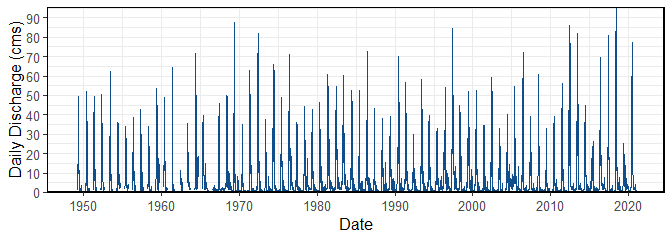When plotting multiple stations, they automatically produce a separate plot for each station. However, setting one_plot = TRUE will plot all stations on the same plot. plot_flow_data(station_number = c("08NM241", "08NM242"), one_plot = TRUE)  $Daily_Flows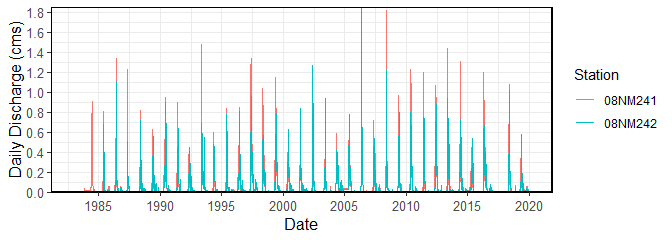To view a flow time series data quality from their provided HYDAT symbols (qualifer symbols like E for estimate, B for under ice etc.), or custom symbols/categories from a column called “Symbol”, the plot_flow_data() function will plot all daily data in the data frame. The plot can be filtered by years and dates.

plot_flow_data_symbols(station_number = "08NM116",
start_year = 1972, end_year = 1976) 
$Flow_Data_Symbols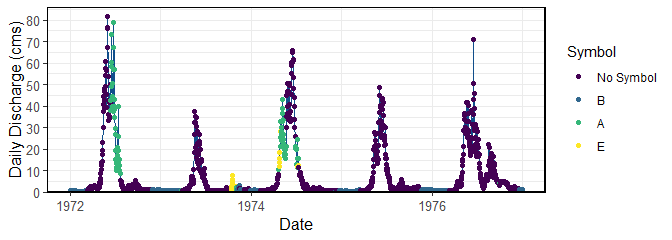The screen_flow_data() function provides an overview of the number of flow values per year and each month per year, along with annual minimums, maximums, means, and standard deviations to inspect for outliers in the data. screen_flow_data(station_number = "08NM116")  STATION_NUMBER Year n_days n_Q n_missing_Q E_Symbol No_Symbol B_Symbol 1 08NM116 1949 365 183 182 10 173 0 2 08NM116 1950 365 183 182 0 183 0 3 08NM116 1951 365 183 182 0 183 0 4 08NM116 1952 366 183 183 0 183 0 5 08NM116 1953 365 183 182 0 183 0 6 08NM116 1954 365 183 182 0 183 0 A_Symbol Minimum Maximum Mean Median StandardDeviation Jan_missing_Q 1 0 0.623 49.3 7.771066 2.27 10.49771 31 2 0 0.623 52.1 7.760432 2.07 10.77839 31 3 0 0.623 49.3 8.991197 3.71 10.91531 31 4 0 0.850 50.7 10.277541 3.17 11.87987 31 5 0 0.340 62.3 8.303328 4.56 9.52511 31 6 0 0.566 36.2 11.281011 5.38 10.96519 31 Feb_missing_Q Mar_missing_Q Apr_missing_Q May_missing_Q Jun_missing_Q 1 28 31 0 0 0 2 28 31 0 0 0 3 28 31 0 0 0 4 29 31 0 0 0 5 28 31 0 0 0 6 28 31 0 0 0 Jul_missing_Q Aug_missing_Q Sep_missing_Q Oct_missing_Q Nov_missing_Q 1 0 0 0 31 30 2 0 0 0 31 30 3 0 0 0 31 30 4 0 0 0 31 30 5 0 0 0 31 30 6 0 0 0 31 30 Dec_missing_Q 1 31 2 31 3 31 4 31 5 31 6 31 To view the summary data in the screen_flow_data() function, the plot_data_screening() function will plot the annual minimums, maximums, means, medians, and standard deviations, with the point coloured by data availability. plot_data_screening(station_number = "08NM116")  $Data_Screening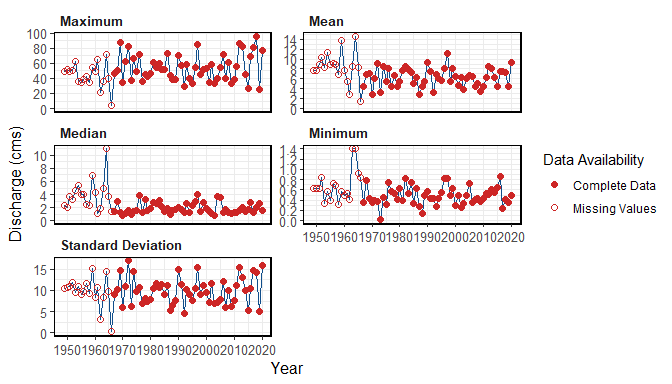Use the plot_missing_dates() function to plot out the missing dates for each month of each year to view for data availability and gaps.

plot_missing_dates(station_number = "08NM116") 
$Missing_Dates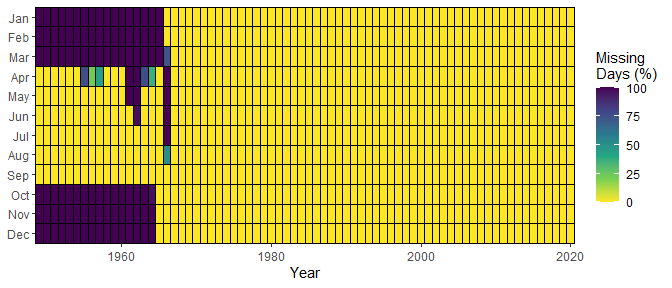Use the plot_annual_symbols() function to plot the symbols on an annual basis to view the data quality and data availability. The default plots by day of year, but there are options to view annual counts of symbols. plot_annual_symbols(station_number = "08NM116")  $Annual_Symbols
Warning: Removed 144 rows containing missing values (geom_tile()).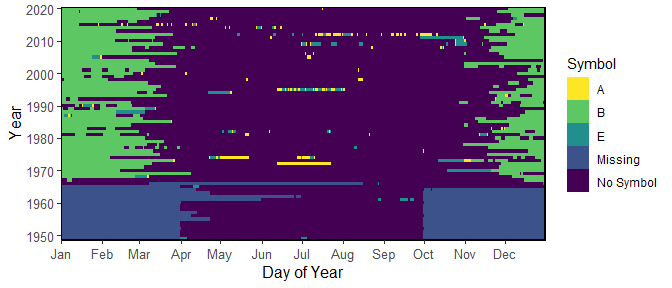## 6. Functions for Calculating Statistics

The majority of the fasstr functions produce statistics over a certain time period, either long-term, annually, monthly, or daily. These statistics are produced using the calc_* functions and can be visualized using their corresponding plot_* functions. The following sections are an overview of these functions.

### Basic Summary Statistics

These functions calculate the means, medians, maximums, minimums, and percentiles (choose using the percentiles argument) of a flow data set:

• calc_longterm_daily_stats() - calculate the long-term and long-term monthly summary statistics based on daily mean flows
• calc_longterm_monthly_stats() - calculate the long-term annual and monthly summary statistics based on monthly mean flows
• calc_annual_stats() - calculate annual summary statistics
• calc_monthly_stats() - calculate annual monthly summary statistics
• calc_daily_stats() - calculate daily summary statistics

These basic statistics can also be viewed using their corresponding plotting functions:

• plot_longterm_daily_stats() - plot the long-term monthly summary statistics based on daily mean flows
• plot_longterm_monthly_stats() - plot the long-term monthly summary statistics based on annual monthly mean flows
• plot_annual_stats() - plot annual summary statistics
• plot_monthly_stats() - plot annual monthly summary statistics
• plot_daily_stats() - plot daily summary statistics

This function produced flow duration curves:

• plot_flow_duration() - plot flow duration curves

These other long-term functions summarize the data over the entire record:

• calc_longterm_mean() - calculate the long-term mean annual discharge
• calc_longterm_percentile() - calculate the long-term percentiles
• calc_flow_percentile() - calculate the percentile rank of a flow value

#### Basic long-term statistics

The long-term calc_ and plot_ functions calculate the long-term and long-term monthly mean, median, maximum, minimum, and percentiles of all daily mean flows.

For calc_longterm_daily_stats(), for a given month, all daily flow values for a given month over the entire record are summarized together. For the ‘Long-term’ category, it summarizes all flow values over the entire record to determine the mean, median, maximum, minimum, and selected percentiles of daily flows. You can also specify a certain period of months to summarize together (ex. Jul-Sep flows) using the custom_months argument (listing the months) and labeling it using the custom_months_label argument (ex. “Summer Flows”).

calc_longterm_daily_stats(station_number = "08NM116",
start_year = 1974)
   STATION_NUMBER     Month      Mean  Median Maximum Minimum     P10    P90
1         08NM116       Jan  1.122294  0.9340    9.50   0.160  0.5706  1.750
2         08NM116       Feb  1.127140  0.9435    5.81   0.140  0.5064  1.900
3         08NM116       Mar  1.748453  1.2400   17.50   0.380  0.6776  3.404
4         08NM116       Apr  8.169997  5.8000   53.50   0.505  1.4200 18.300
5         08NM116       May 25.182745 22.4000   95.40   2.550 10.4000 44.340
6         08NM116       Jun 22.375935 19.9000   86.20   0.450  6.1390 40.800
7         08NM116       Jul  6.237522  3.9700   76.80   0.332  1.2200 13.900
8         08NM116       Aug  2.176655  1.6300   22.40   0.427  0.8466  4.008
9         08NM116       Sep  2.319518  1.6300   17.60   0.364  0.7859  4.751
10        08NM116       Oct  2.152216  1.7200   15.20   0.267  0.8576  4.122
11        08NM116       Nov  1.950852  1.5600   11.70   0.260  0.6078  3.741
12        08NM116       Dec  1.254868  1.0700    7.30   0.244  0.5468  2.150
13        08NM116 Long-term  6.331062  1.8200   95.40   0.140  0.7060 20.100

The plot_longterm_daily_stats() will plot the monthly mean, median, maximum, and minimum values along with selected inner and outer percentiles ribbons on one plot. Change the inner and outer percentile ranges using the inner_percentiles and outer_percentiles arguments, remove the maximum and minimum ribbon using include_extremes = FALSE, or add a specific year using add_year.

plot_longterm_daily_stats(station_number = "08NM116",
start_year = 1974,
inner_percentiles = c(25,75),
outer_percentiles = c(10,90)) 
$Long-term_Daily_Statistics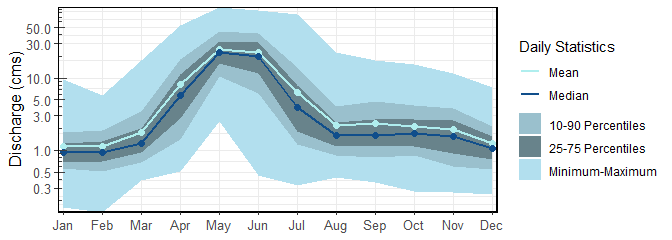Similarly, the calc_longterm_monthly_stats() functions will calculate the mean, median, maximum, and percentiles of monthly mean flows from all years. Meaning the all daily flows for each month and each year are averaged, and the statistics are based on these annual monthly means. The “Annual” data row summarizes the mean, median, maximum, and percentiles from all annual means. calc_longterm_monthly_stats(station_number = "08NM116", start_year = 1974)  STATION_NUMBER Month Mean Median Maximum Minimum P10 1 08NM116 Jan 1.122294 0.9679032 6.117742 0.3155161 0.6246516 2 08NM116 Feb 1.128056 0.9607586 3.831786 0.3528276 0.5149286 3 08NM116 Mar 1.748453 1.4009677 6.926774 0.5067419 0.8168000 4 08NM116 Apr 8.169997 7.7613333 23.880333 1.5993333 2.9978667 5 08NM116 May 25.182745 23.8483871 48.122581 13.9861288 16.1646452 6 08NM116 Jun 22.375935 21.8166669 48.640000 3.1504333 11.3063333 7 08NM116 Jul 6.237522 4.5012903 25.639355 0.9213871 2.0231935 8 08NM116 Aug 2.176655 1.7938710 10.193548 0.8721290 1.1316645 9 08NM116 Sep 2.319518 1.7580000 8.109333 0.6999667 1.0131933 10 08NM116 Oct 2.152216 1.8951613 5.661290 0.5329032 1.0350516 11 08NM116 Nov 1.950852 1.5526667 5.413667 0.4982333 0.7192933 12 08NM116 Dec 1.254868 1.0968065 3.648387 0.4502581 0.5574323 13 08NM116 Annual 6.330833 6.2744794 11.134121 2.8761370 4.3721797 P90 1 1.643419 2 1.696507 3 2.764129 4 12.821133 5 33.928387 6 36.802000 7 12.742839 8 3.365161 9 3.991000 10 3.628335 11 3.316533 12 2.093226 13 8.441362 The corresponding plot_longterm_monthly_stats() function plots the data, with similar options as plot_longterm_daily_stats(). plot_longterm_monthly_stats(station_number = "08NM116", start_year = 1974)  $Long-term_Monthly_Statistics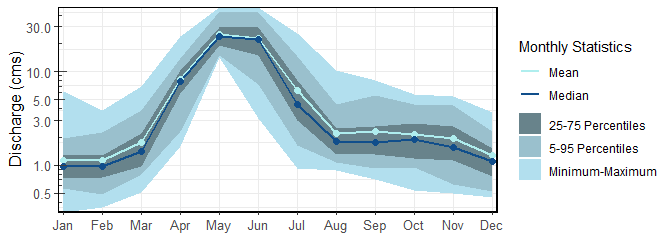#### Basic annual statistics

The calc_annual_stats() and plot_annual_stats() functions calculate the mean, median, maximum, minimum, and percentiles of daily flows for every year of data provided. In calculating, all daily flow values are grouped by year.

calc_annual_stats(station_number = "08NM116",
start_year = 1974)
  STATION_NUMBER Year     Mean Median Maximum Minimum    P10   P90
1        08NM116 1974 8.430181   1.34    66.0   0.447 0.7092 32.98
2        08NM116 1975 5.482636   1.54    48.7   0.320 0.5800 19.58
3        08NM116 1976 8.180694   3.84    71.1   0.736 0.8835 25.55
4        08NM116 1977 4.381567   1.26    36.0   0.564 0.7760 17.20
5        08NM116 1978 6.747608   3.28    44.5   0.532 0.8278 19.70
6        08NM116 1979 4.401564   1.56    43.0   0.411 0.6182 15.88

The percentiles in the plot_annual_stats() function are fully customizable like the calc_ function.

plot_annual_stats(station_number = "08NM116",
start_year = 1974,
log_discharge = TRUE) 
$Annual_Statistics Warning: Transformation introduced infinite values in continuous y-axis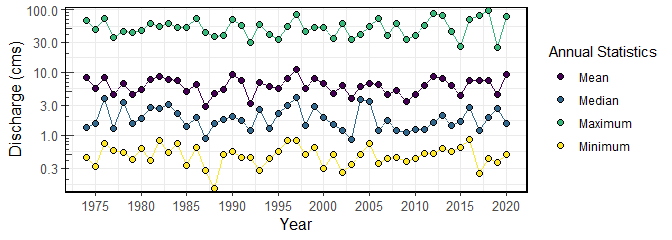#### Basic monthly statistics The calc_monthly_stats() and plot_monthly_stats() functions calculate the mean, median, maximum, minimum, and percentiles of daily flows for each month of each year. In calculating, all daily flow values are grouped by year and month. calc_monthly_stats(station_number = "08NM116", start_year = 1974)  STATION_NUMBER Year Month Mean Median Maximum Minimum P10 P90 1 08NM116 1974 Jan 1.0234194 1.020 1.26 0.864 0.9060 1.120 2 08NM116 1974 Feb 0.9848214 0.984 1.06 0.830 0.9442 1.043 3 08NM116 1974 Mar 1.2113226 1.120 2.14 0.855 0.9370 1.970 4 08NM116 1974 Apr 7.7613333 4.910 28.30 1.850 1.9190 18.680 5 08NM116 1974 May 29.8451611 30.300 50.40 15.900 17.5000 43.300 6 08NM116 1974 Jun 44.4600002 44.900 66.00 20.600 25.6100 61.250 The percentiles in the plot_monthly_stats() function are fully customizable like the calc_ function. A plot for each different statistic (means, medians, percentiles, etc.) is created to visualize the monthly patterns over the years. plot_monthly_stats(station_number = "08NM116", start_year = 1974) $Mean_Monthly_Statistics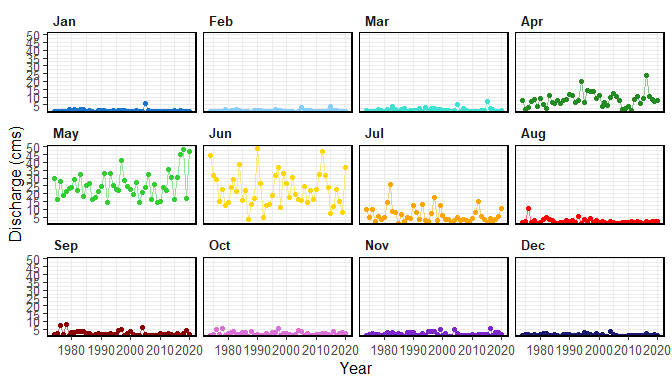#### Basic daily statistics

The calc_daily_stats() and plot_daily_stats() functions calculate the mean, median, maximum, minimum, and percentiles of daily flows for each day of the year. For example, for a given day of year (i.e. day 1 (Jan-01) or day 2 (Jan-02)), all flow values for that day from the entire record are summarized together. Only the first 365 days of each year are summarized (ignores the 366th day from leap years). In calculating, all daily flow values are grouped by day of year.

calc_daily_stats(station_number = "08NM116",
start_year = 1974)
  STATION_NUMBER   Date DayofYear     Mean Median Minimum Maximum     P5    P25
1        08NM116 Jan-01         1 1.070319  0.977   0.328    2.51 0.5455 0.6850
2        08NM116 Jan-02         2 1.044745  0.929   0.310    2.26 0.5320 0.6795
3        08NM116 Jan-03         3 1.024426  0.905   0.290    2.00 0.5290 0.7020
4        08NM116 Jan-04         4 1.035596  0.910   0.284    2.52 0.5113 0.7235
5        08NM116 Jan-05         5 1.021064  0.899   0.302    2.25 0.5390 0.7090
6        08NM116 Jan-06         6 1.015681  0.870   0.315    2.32 0.5201 0.7335
P75   P95
1 1.300 1.850
2 1.300 1.870
3 1.195 1.927
4 1.215 1.905
5 1.190 1.929
6 1.250 1.871

The plotting daily statistics function will plot the monthly mean, median, maximum, and minimum values along with selected inner and outer percentiles ribbons on one plot. Change the inner and outer percentile ranges using the inner_percentiles and outer_percentiles arguments, remove the maximum and minimum ribbon using include_extremes = FALSE, or add a specific year using add_year.

plot_daily_stats(station_number = "08NM116",
start_year = 1974) 
$Daily_Statistics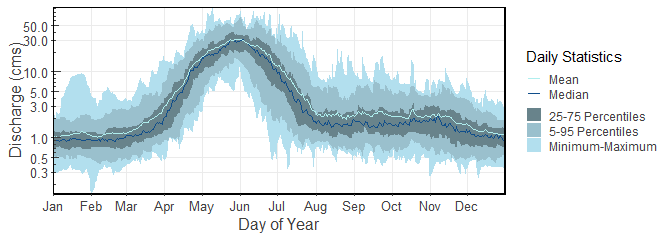plot_daily_stats(station_number = "08NM116", start_year = 1974, add_year = 2000)  $Daily_Statistics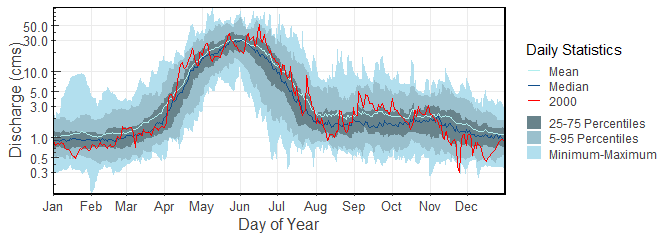#### Flow Duration

Flow duration curves can be produced using the function, where selected months and time periods can be selected:

plot_flow_duration(station_number = "08NM116",
start_year = 1974) 
$Flow_Duration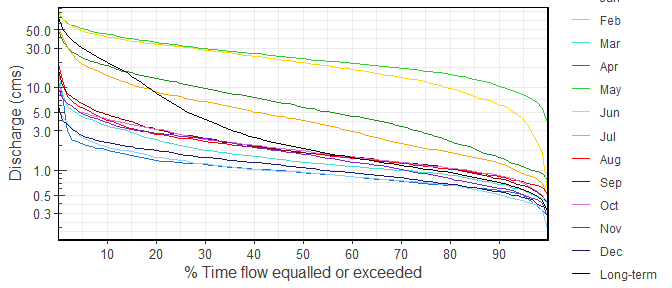plot_flow_duration(station_number = "08NM116", start_year = 1974, months = 7:9, include_longterm = FALSE)  $Flow_Duration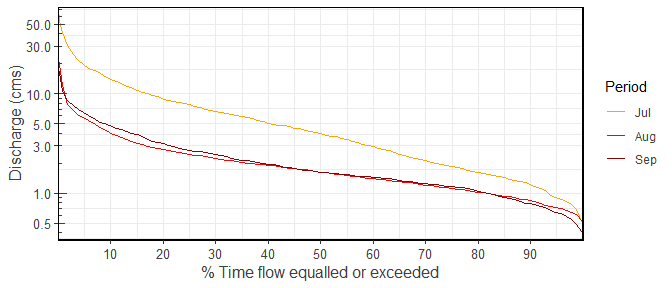#### Other Long-term Statistics

calc_longterm_mean() calculates the mean of all the daily flows, and specific percents of the long-term mean (using percent_MAD argument). It can also be known as the long-term mean annual discharge, MAD.

calc_longterm_mean(station_number = "08NM116",
start_year = 1974,
percent_MAD = c(5,10,20))
  STATION_NUMBER    LTMAD    X5.MAD   X10.MAD  X20.MAD
1        08NM116 6.331062 0.3165531 0.6331062 1.266212

calc_longterm_percentile() calculates the selected long-term percentiles of all the daily flow values.

calc_longterm_percentile(station_number = "08NM116",
start_year = 1974,
percentiles = c(25,50,75))
  STATION_NUMBER  P25  P50  P75
1        08NM116 1.03 1.82 5.67

calc_flow_percentile() calculates the percentile rank of a specified flow value, provided as flow_value. It compares the flow value to all daily flow values to determines the percentile rank.

calc_flow_percentile(station_number = "08NM116",
start_year = 1974,
flow_value = 6.270)
  STATION_NUMBER Percentile
1        08NM116     76.397

#### Basic statistics and plotting volumetric and yield flows

The calc_ and plot_ functions will summarize any values provided to the functions with the default column being ‘Value’. While for fasstr this defaults to daily mean flows, any daily value can be summarized (water level, precipitation amount, etc.) if the methods of analyses are similar for the parameter type. As there are no units presented in the calc_ functions this should not be problem for most calculations. However, the plots come standard with a “Discharge (cms)” y-axis, which can be changed afterwards using ggplot2 functions.

To facilitate the plotting of the daily volume or yield statistics from fasstr, after adding them to your flow data using the add_daily_volume() or add_daily_yield() functions, by listing the values argument as either ‘Volume_m3’ or ‘Yield_mm’ (from their respective add_* functions), the discharge axis title will adjust accordingly.

add_daily_volume(station_number = "08NM116") %>%
plot_annual_stats(values = "Volume_m3",
start_year = 1974) 
$Annual_Statistics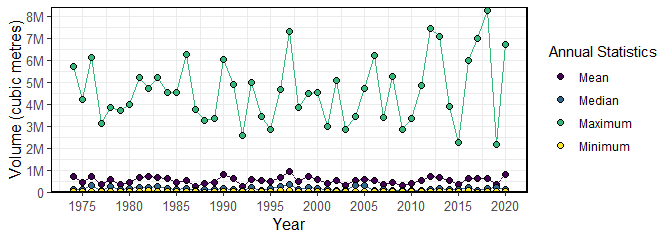add_daily_yield(station_number = "08NM116") %>% plot_daily_stats(values = "Yield_mm", start_year = 1974)  $Daily_Statistics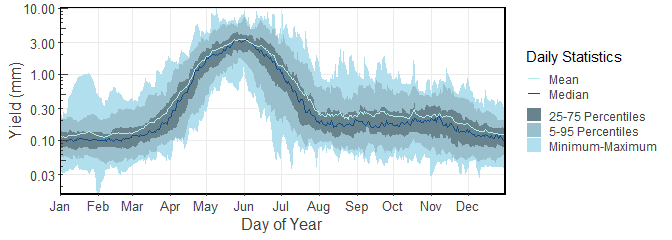### Cumulative Flow Statistics

Total volumetric of runoff yield flows within a given year can provide important hydrological information on a basin-wide scale. These functions calculate the total volume (in cubic metres) or yield (in millimetres; based on basin size) for a flow data set, at the annual, monthly, or daily cumulative scale.

• calc_annual_cumulative_stats() - calculate annual (and seasonal) cumulative flows
• calc_monthly_cumulative_stats() - calculate cumulative monthly flow statistics
• calc_daily_cumulative_stats() - calculate cumulative daily flow statistics

These statistics can also be viewed using their corresponding plotting functions:

• plot_annual_cumulative_stats() - plot annual and seasonal total flows
• plot_monthly_cumulative_stats() - plot cumulative monthly flow statistics
• plot_daily_cumulative_stats() - plot cumulative daily flow statistics

While these functions default to volumetric flows, using use_yield = TRUE and basin_area arguments will calculate totals in runoff yield. If there is a groups column of HYDAT station numbers, then the function will automatically pull the basin area out of HYDAT if available; otherwise a basin area will be required. Due to the requirements of a complete annual data set to calculate total flows, only years of complete data are used.

#### Cumulative annual statistics

The calc_annual_cumulative_stats() function provides the total annual volume or runoff yield (if use_yield = TRUE is used). It totals all flows for a given year in cubic metres.

calc_annual_cumulative_stats(station_number = "08NM116", start_year = 1974)
  STATION_NUMBER Year Total_Volume_m3
1        08NM116 1974       265854182
2        08NM116 1975       172900397
3        08NM116 1976       258693177
4        08NM116 1977       138177100
5        08NM116 1978       212792574
6        08NM116 1979       138807734

By using the include_seasons = TRUE (logical TRUE/FALSE) argument, total seasonal flows columns will be added to the results. Two columns of two-seasons (2-six months), and four columns of four-seasons (4-three months) will be added. The start month of the first seasons will begin in the first month of the year (ex. Jan for Calendar years or Oct for water years starting in October).

calc_annual_cumulative_stats(station_number = "08NM116",
start_year = 1974,
include_seasons = TRUE)
  STATION_NUMBER Year Total_Volume_m3 Jan.Jun_Volume_m3 Jul.Dec_Volume_m3
1        08NM116 1974       265854182         223662989          42191194
2        08NM116 1975       172900397         136045958          36854438
3        08NM116 1976       258693177         164417817          94275360
4        08NM116 1977       138177100         115279113          22897987
5        08NM116 1978       212792574         146659335          66133239
6        08NM116 1979       138807734         117444383          21363350
Jan.Mar_Volume_m3 Apr.Jun_Volume_m3 Jul.Sep_Volume_m3 Oct.Dec_Volume_m3
1           8368013         215294976          35216640           6974554
2           5258218         130787741          24657350          12197088
3           7154266         157263552          72438624          21836736
4           6635779         108643334          13898650           8999338
5           9063014         137596321          42360192          23773046
6          13535424         103908959          14050541           7312810

The total volumes for each year can be plotted using the plot_annual_cumulative_stats() function. When using include_seasons = TRUE two additional plots will be created, one for two- and four-seasons.

plot_annual_cumulative_stats(station_number = "08NM116",
start_year = 1974) 
$Total_Volume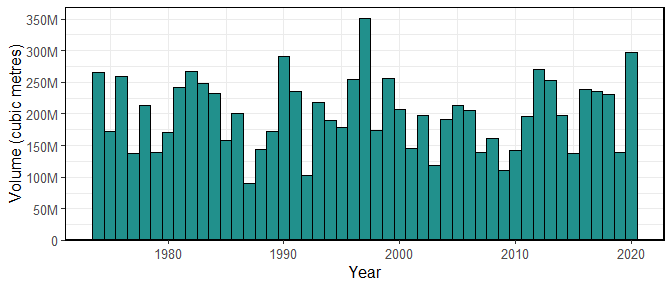#### Cumulative monthly and statistics The calc_monthly_cumulative_stats() and plot_monthly_cumulative_stats() functions calculate the mean, median, maximum, minimum, and percentiles of total cumulative monthly flows. For each month of each year, the total volume or runoff yield is determined. Then within a given year, the cumulative total for each month is determined by added all previous months (ex. Jan = Jan total; Feb = Jan+Feb totals, etc.). Then the mean, median, maximum, minimum, and percentiles are calculated based on those monthly cumulative totals for each year. In interpreting the information, if a given total flow is below the mean value, then the cumulative flow is less than average, or less volume has passed through the station than average at that point in time. The percentiles in the calc_ function are flexible using the percentiles argument. calc_monthly_cumulative_stats(station_number = "08NM116", start_year = 1974)  STATION_NUMBER Month Mean Median Maximum Minimum P5 1 08NM116 Jan 3005952 2592432 16385760 845078.4 1503135 2 08NM116 Feb 5757593 4928947 24560928 1729123.2 2724589 3 08NM116 Mar 10440650 8677498 38265696 3086380.8 4902707 4 08NM116 Apr 31617282 28485389 74097331 9895046.4 13125819 5 08NM116 May 99066747 93268887 159751008 50343551.6 54632431 6 08NM116 Jun 157065172 158564131 255162529 76246877.0 90921640 7 08NM116 Jul 173771752 177670628 301884193 81422928.3 99999127 8 08NM116 Aug 179601706 181262189 311904865 84962822.7 103871315 9 08NM116 Sep 185613898 188800847 323685505 86777136.3 106433697 10 08NM116 Oct 191378392 192925325 337755745 88204464.3 109851699 11 08NM116 Nov 196435000 196449148 346120993 89495885.1 111684986 12 08NM116 Dec 199796039 197871983 351125627 90701856.3 113122665 P25 P75 P95 1 1950221 3407616 5231434 2 3743410 6122650 10551946 3 6587179 11744741 20279117 4 24031642 36248083 63880462 5 77104137 117601934 155839473 6 123343214 185879578 228437522 7 128909577 208896667 259840380 8 132292699 219312101 265726294 9 137414966 228368981 270057785 10 143054726 231213701 274458404 11 148326379 235173715 280909365 12 152096011 239892883 284372018 The plot_monthly_cumulative_stats() function will plot the monthly total mean, median, maximum, and minimum values along with the 5th, 25th, 75th, and 95th percentiles all on one plot. The percentiles are not customizable for this function. plot_monthly_cumulative_stats(station_number = "08NM116", start_year = 1974)  $Monthly_Cumulative_Volumetric_Stats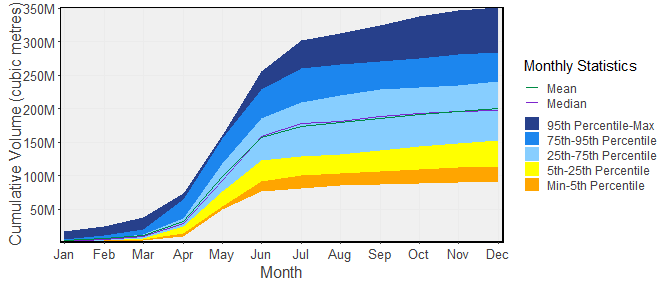#### Cumulative daily statistics

The calc_daily_cumulative_stats() and plot_daily_cumulative_stats() functions calculate the mean, median, maximum, minimum, and percentiles of total cumulative daily flows. For each day of each year, the total volume or runoff yield is determined. Then within a given year, the cumulative total for each day is determined by added all previous days (ex. Jan-01 = Jan-01 total; Jan-02 = Jan-01+Jan-02 totals, etc.). Then the mean, median, maximum, minimum, and percentiles are calculated based on those daily cumulative totals for each year. In interpreting the information, if a given total flow is below the mean value, then the cumulative flow is less than average. In other words, less volume has passed through the station than normal at that point in time. Viewing the plot below may help understand how this function works. The percentiles in the calc_ function are flexible using the percentiles argument.

calc_daily_cumulative_stats(station_number = "08NM116",
start_year = 1974)
  STATION_NUMBER   Date DayofYear      Mean   Median  Minimum Maximum       P5
1        08NM116 Jan-01         1  92475.57  84412.8  28339.2  216864  47131.2
2        08NM116 Jan-02         2 182741.51 164678.4  55123.2  412128  92793.6
3        08NM116 Jan-03         3 271251.88 241488.0  80179.2  581472 136987.2
4        08NM116 Jan-04         4 360727.35 316137.6 104716.8  768960 183902.4
5        08NM116 Jan-05         5 448947.27 391478.4 130809.6  952992 233496.0
6        08NM116 Jan-06         6 536702.09 474681.6 158025.6 1123200 282398.4
P25      P75      P95
1  59184.0 112320.0 159840.0
2 117892.8 220320.0 325209.6
3 176083.2 331344.0 482544.0
4 239932.8 430272.0 621216.0
5 307497.6 531792.0 778377.6
6 367113.6 636163.2 958867.2

The plot_daily_cumulative_stats() function will plot the daily cumulative total mean, median, maximum, and minimum values along with the 5th, 25th, 75th, and 95th percentiles all on one plot. The percentiles are not customizable for this function.

plot_daily_cumulative_stats(station_number = "08NM116",
start_year = 1974,
use_yield = TRUE) 
$Daily_Cumulative_Yield_Stats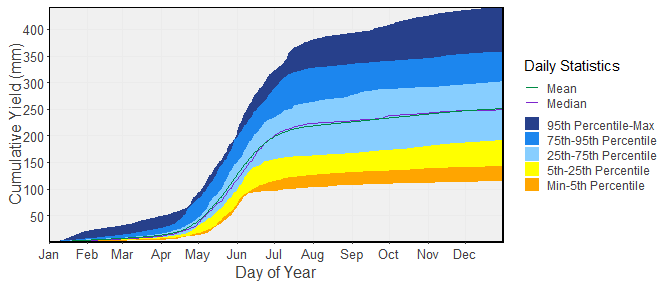### Other Annual Statistics Beside the basic summary statistics, there are other useful statistics for interpreting annual streamflow data. They include the following:: • calc_annual_flow_timing() - calculate annual flow timing • calc_annual_lowflows() - calculate multiple n-day annual low flow values and dates • calc_annual_highflows() - calculate multiple n-day annual high flow values and dates • calc_annual_extremes() - calculate annual low and high flow values and dates • calc_annual_normal_days() - calculate annual normal days and days above and below normal • calc_all_annual_stats() - calculate all fasstr annual statistics and their corresponding and other plotting functions: • plot_annual_flow_timing() - plot annual flow timing • plot_annual_lowflows() - plot multiple n-day annual low flow values and dates • plot_annual_highflows() - plot multiple n-day annual low flow values and dates • plot_annual_extremes() - plot annual low and high flow values and dates • plot_annual_normal_days() - plot annual normal days and days above and below normal • plot_annual_means() - plot annual means compared to the long-term mean There are also a few functions that view give some of the annual statistics context: • plot_annual_flow_timing_year() - plot annual flow timing for a given year • plot_annual_extremes_year() - plot annual low and high flow values and dates for a given year • plot_annual_normal_days_year() - plot annual normal days and days above and below normal for a given year #### Annual flow timing The calc_annual_flow_timing() calculates the day of year when a portion of a total annual volumetric flow has occurred. Using the percent_total argument, one or multiple portions of annual flow can be calculated. Using 50 as the percent_total is similar to the center of volume or timing of half flow. The day of year and date will be also be produced. calc_annual_flow_timing(station_number = "08NM116", start_year = 1974)  STATION_NUMBER Year DoY_25pct_TotalQ Date_25pct_TotalQ DoY_33.3pct_TotalQ 1 08NM116 1974 135 1974-05-15 146 2 08NM116 1975 146 1975-05-26 153 3 08NM116 1976 143 1976-05-22 151 4 08NM116 1977 124 1977-05-04 131 5 08NM116 1978 134 1978-05-14 142 6 08NM116 1979 126 1979-05-06 133 Date_33.3pct_TotalQ DoY_50pct_TotalQ Date_50pct_TotalQ DoY_75pct_TotalQ 1 1974-05-26 158 1974-06-07 173 2 1975-06-02 162 1975-06-11 177 3 1976-05-30 169 1976-06-17 220 4 1977-05-11 147 1977-05-27 165 5 1978-05-22 158 1978-06-07 204 6 1979-05-13 145 1979-05-25 161 Date_75pct_TotalQ 1 1974-06-22 2 1975-06-26 3 1976-08-07 4 1977-06-14 5 1978-07-23 6 1979-06-10 The timing of flows can also be plotted. plot_annual_flow_timing(station_number = "08NM116", start_year = 1974)  $Annual_Flow_Timing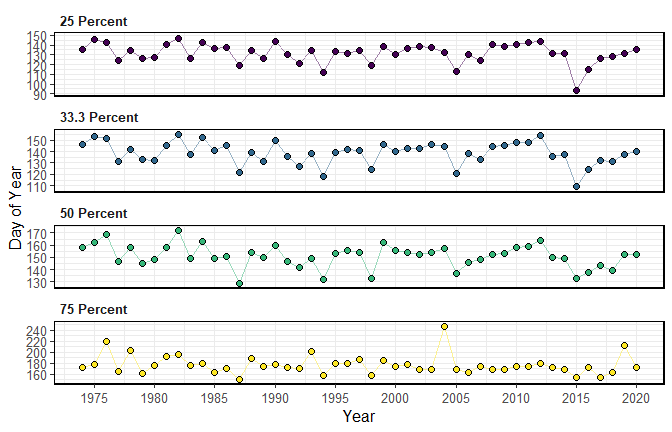The timing of flows for a given year can also be plotted.

plot_annual_flow_timing_year(station_number = "08NM116",
year_to_plot = 1999) 
Warning: One or more calculations included missing values and NA's were
produced. If desired, filter data for complete years or months, or use the
'ignore_missing' or 'allowed_missing' arguments (if applicable) to ignore or
allow some missing values.
$Annual_Flow_Timing_Year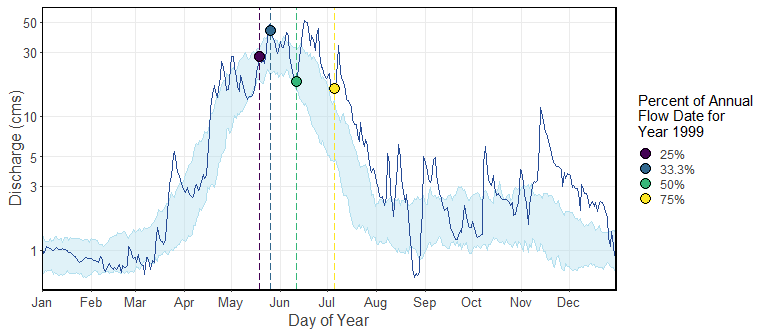#### Annual low-flows The calc_annual_lowflows() calculates the annual minimum values, the day of year, and dates of specified rolling mean days (can do multiple days if desired). calc_annual_lowflows(station_number = "08NM116", start_year = 1974)  STATION_NUMBER Year Min_1_Day Min_1_Day_DoY Min_1_Day_Date Min_3_Day 1 08NM116 1974 0.447 333 1974-11-29 0.5333333 2 08NM116 1975 0.320 11 1975-01-11 0.3783333 3 08NM116 1976 0.736 38 1976-02-07 0.7406667 4 08NM116 1977 0.564 73 1977-03-14 0.6273333 5 08NM116 1978 0.532 55 1978-02-24 0.6296667 6 08NM116 1979 0.411 268 1979-09-25 0.4156667 Min_3_Day_DoY Min_3_Day_Date Min_7_Day Min_7_Day_DoY Min_7_Day_Date 1 334 1974-11-30 0.6018572 346 1974-12-12 2 39 1975-02-08 0.4158571 41 1975-02-10 3 63 1976-03-03 0.7564286 65 1976-03-05 4 252 1977-09-09 0.6865714 79 1977-03-20 5 3 1978-01-03 0.6642857 5 1978-01-05 6 269 1979-09-26 0.4370000 270 1979-09-27 Min_30_Day Min_30_Day_DoY Min_30_Day_Date 1 0.6645667 358 1974-12-24 2 0.4937667 58 1975-02-27 3 0.7988333 66 1976-03-06 4 0.7876667 81 1977-03-22 5 0.7551000 16 1978-01-16 6 0.5684333 287 1979-10-14 The annual low flow values and the day of the low flow values can be plotted, separately, using the plot_annual_lowflows() function. plot_annual_lowflows(station_number = "08NM116", start_year = 1974)  $Annual_Low_Flows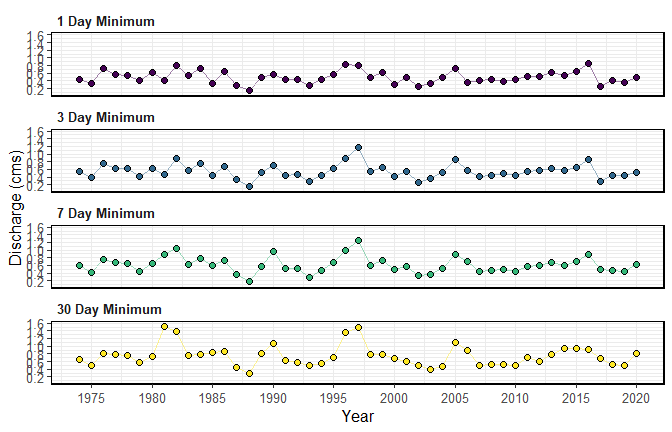$Annual_Low_Flows_Dates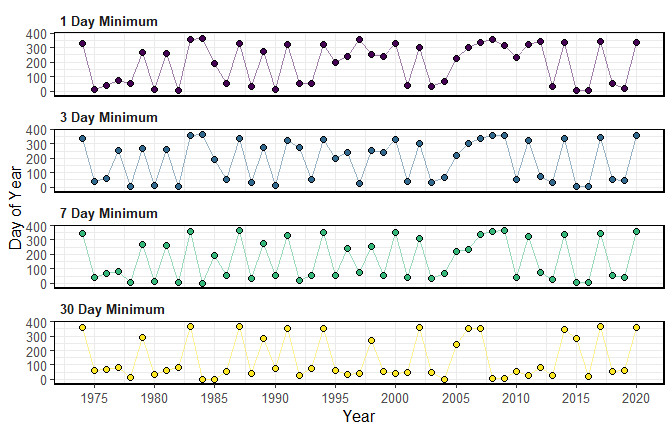#### Annual high flows The calc_annual_highflows() calculates the annual maximum values, the day of year, and dates of specified rolling mean days (can do multiple days if desired). calc_annual_highflows(station_number = "08NM116", start_year = 1974)  STATION_NUMBER Year Max_1_Day Max_1_Day_DoY Max_1_Day_Date Max_3_Day 1 08NM116 1974 66.0 168 1974-06-17 64.26667 2 08NM116 1975 48.7 153 1975-06-02 47.46667 3 08NM116 1976 71.1 168 1976-06-16 54.93333 4 08NM116 1977 36.0 123 1977-05-03 33.00000 5 08NM116 1978 44.5 157 1978-06-06 42.66667 6 08NM116 1979 43.0 147 1979-05-27 40.16667 Max_3_Day_DoY Max_3_Day_Date Max_7_Day Max_7_Day_DoY Max_7_Day_Date 1 170 1974-06-19 62.21429 171 1974-06-20 2 155 1975-06-04 41.85714 158 1975-06-07 3 170 1976-06-18 45.02857 173 1976-06-21 4 159 1977-06-08 27.11429 161 1977-06-10 5 157 1978-06-06 37.01429 159 1978-06-08 6 147 1979-05-27 34.15714 148 1979-05-28 Max_30_Day Max_30_Day_DoY Max_30_Day_Date 1 47.63000 174 1974-06-23 2 33.07667 178 1975-06-27 3 31.15000 173 1976-06-21 4 20.15000 160 1977-06-09 5 26.74333 166 1978-06-15 6 23.97000 154 1979-06-03 The annual high flow values and the day of the high flow values can be plotted, separately, using the plot_annual_highflows() function. plot_annual_highflows(station_number = "08NM116", start_year = 1974)  $Annual_High_Flows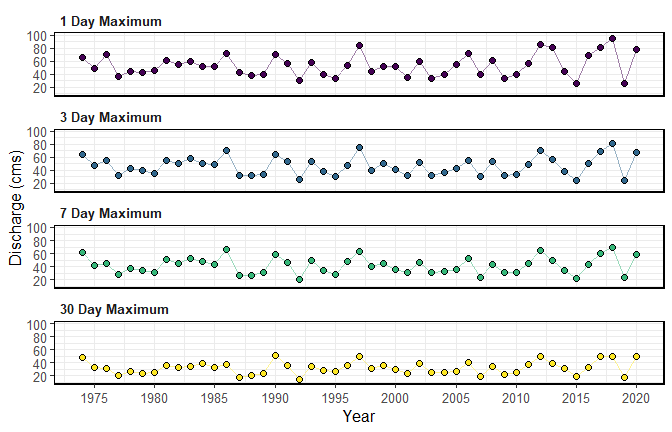$Annual_High_Flows_Dates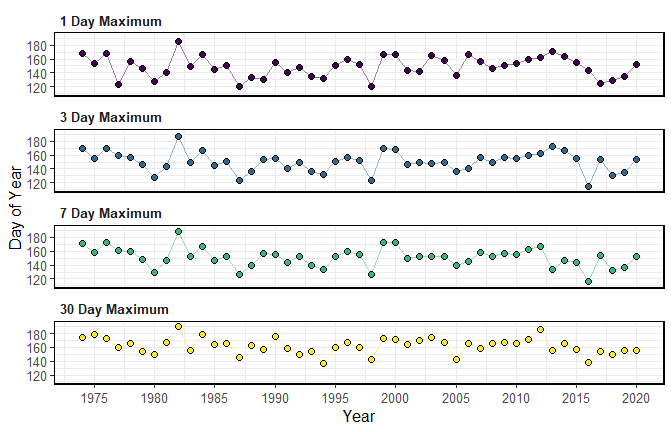#### Annual extreme (both high and low) flows Similar to *_annual_lowflows() and *_annual_highflows(), calc_annual_extremes() calculates the annual maximum and minimum values, the day of year, and dates of specified rolling mean days and specified months for each of the high and low flows. calc_annual_extremes(station_number = "08NM116", roll_days_min = 7, roll_days_max = 3, start_year = 1974)  STATION_NUMBER Year Min_7_Day Min_7_Day_DoY Min_7_Day_Date Max_3_Day 1 08NM116 1974 0.6018572 346 1974-12-12 64.26667 2 08NM116 1975 0.4158571 41 1975-02-10 47.46667 3 08NM116 1976 0.7564286 65 1976-03-05 54.93333 4 08NM116 1977 0.6865714 79 1977-03-20 33.00000 5 08NM116 1978 0.6642857 5 1978-01-05 42.66667 6 08NM116 1979 0.4370000 270 1979-09-27 40.16667 Max_3_Day_DoY Max_3_Day_Date 1 170 1974-06-19 2 155 1975-06-04 3 170 1976-06-18 4 159 1977-06-08 5 157 1978-06-06 6 147 1979-05-27 The annual extremes values and the days can be plotted: plot_annual_extremes(station_number = "08NM116", roll_days_min = 7, roll_days_max = 3, start_year = 1974) $Annual_Extreme_Flows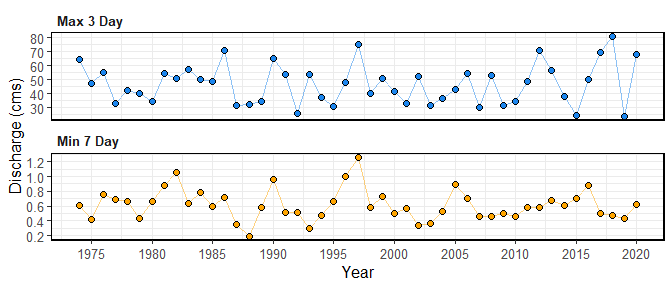$Annual_Extreme_Flows_Dates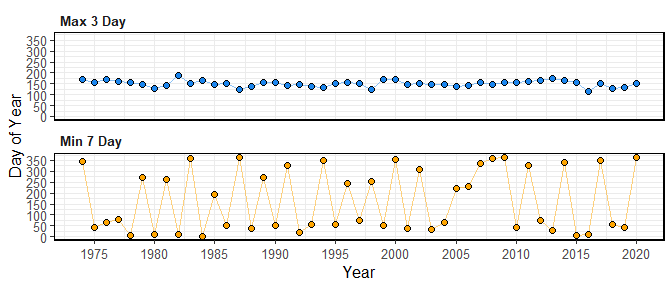The annual extremes values and the days for a given year can also be plotted: plot_annual_extremes_year(station_number = "08NM116", roll_days_min = 7, roll_days_max = 3, start_year = 1974, year_to_plot = 1999) $Annual_Extremes_Year
Warning: Transformation introduced infinite values in continuous y-axis
Transformation introduced infinite values in continuous y-axis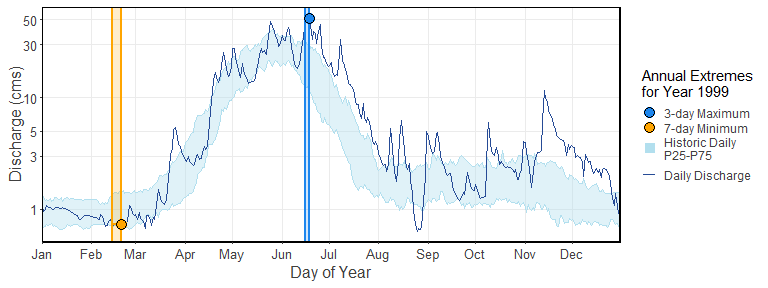#### Number of normal (and above/below normal) days per year

The calc_annual_normal_days() calculates the number of days per year that are normal and above and below “normal”, “normal” typically defined as 25th and 75th percentiles. The normal limits can be determined using the normal_percentiles argument, listing the lower and upper normal ranges, respectively (e.g. normal_percentiles = c(25, 75)). The function calculates the lower and upper percentiles for each day of the year over all years and sums all days that are within and above or below the daily normal ranges for a given year. Rolling averages can also be used in this function using the roll_days argument.

calc_annual_normal_days(station_number = "08NM116",
start_year = 1974)
  STATION_NUMBER Year Normal_Days Below_Normal_Days Above_Normal_Days
1        08NM116 1974         209                78                78
2        08NM116 1975         196               136                33
3        08NM116 1976         168                51               147
4        08NM116 1977         250               106                 9
5        08NM116 1978         236                17               112
6        08NM116 1979         139               155                71

Each of the above, below, and normal days can be plotted using the plot_annual_normal_days() function.

plot_annual_normal_days(station_number = "08NM116",
start_year = 1974) 
$Annual_Normal_Days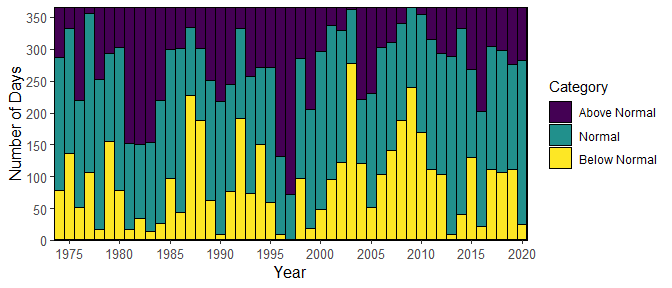The daily flows with normal categories for a given year can also be plotted. plot_annual_normal_days_year(station_number = "08NM116", year_to_plot = 1999)  $Annual_Normal_Days_Year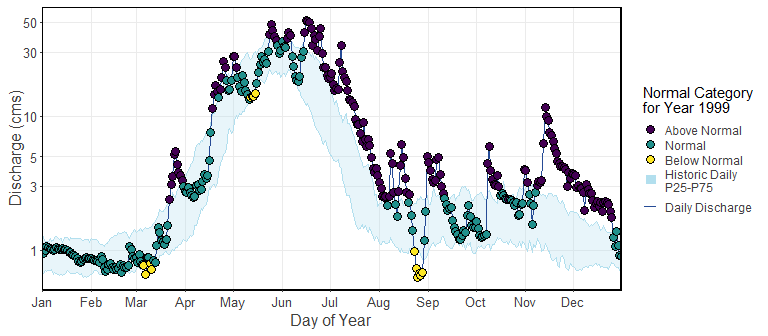#### Calculating all annual statistics

The calc_all_annual_stats() calculates all statistics that have a single annual value. This includes all the calc_annual_* and the calc_monthly_statistics() functions. Several arguments provided for customization of the statistics. There is no corresponding plotting function for this calculation function.

colnames(calc_all_annual_stats(station_number = "08NM116",
start_year = 1974))
   "STATION_NUMBER"    "Year"              "Annual_Maximum"
 "Annual_Mean"       "Annual_Median"     "Annual_Minimum"
 "Annual_P10"        "Annual_P90"        "Min_1_Day"
 "Min_1_Day_DoY"     "Min_3_Day"         "Min_3_Day_DoY"
 "Min_7_Day"         "Min_7_Day_DoY"     "Min_30_Day"
 "Min_30_Day_DoY"    "Total_Volume_m3"   "Jan-Jun_Volume_m3"
 "Jul-Dec_Volume_m3" "Jan-Mar_Volume_m3" "Apr-Jun_Volume_m3"
 "Jul-Sep_Volume_m3" "Oct-Dec_Volume_m3" "Total_Yield_mm"
 "Jan-Jun_Yield_mm"  "Jul-Dec_Yield_mm"  "Jan-Mar_Yield_mm"
 "Apr-Jun_Yield_mm"  "Jul-Sep_Yield_mm"  "Oct-Dec_Yield_mm"
 "DoY_25pct_TotalQ"  "DoY_33pct_TotalQ"  "DoY_50pct_TotalQ"
 "DoY_75pct_TotalQ"  "Normal_Days"       "Below_Normal_Days"
 "Above_Normal_Days" "Jan_Mean"          "Jan_Median"
 "Jan_Maximum"       "Jan_Minimum"       "Jan_P10"
 "Jan_P20"           "Feb_Mean"          "Feb_Median"
 "Feb_Maximum"       "Feb_Minimum"       "Feb_P10"
 "Feb_P20"           "Mar_Mean"          "Mar_Median"
 "Mar_Maximum"       "Mar_Minimum"       "Mar_P10"
 "Mar_P20"           "Apr_Mean"          "Apr_Median"
 "Apr_Maximum"       "Apr_Minimum"       "Apr_P10"
 "Apr_P20"           "May_Mean"          "May_Median"
 "May_Maximum"       "May_Minimum"       "May_P10"
 "May_P20"           "Jun_Mean"          "Jun_Median"
 "Jun_Maximum"       "Jun_Minimum"       "Jun_P10"
 "Jun_P20"           "Jul_Mean"          "Jul_Median"
 "Jul_Maximum"       "Jul_Minimum"       "Jul_P10"
 "Jul_P20"           "Aug_Mean"          "Aug_Median"
 "Aug_Maximum"       "Aug_Minimum"       "Aug_P10"
 "Aug_P20"           "Sep_Mean"          "Sep_Median"
 "Sep_Maximum"       "Sep_Minimum"       "Sep_P10"
 "Sep_P20"           "Oct_Mean"          "Oct_Median"
 "Oct_Maximum"       "Oct_Minimum"       "Oct_P10"
 "Oct_P20"           "Nov_Mean"          "Nov_Median"
 "Nov_Maximum"       "Nov_Minimum"       "Nov_P10"
 "Nov_P20"           "Dec_Mean"          "Dec_Median"
 "Dec_Maximum"       "Dec_Minimum"       "Dec_P10"
 "Dec_P20"          

#### Plotting annual means

The plot_annual_means() function provides a way to visualize how annual means fluctuate around the long-term mean. The x-axis is located at the long-term mean annual discharge (mean of all discharge values over all years) and the bars shows the annual means. The plot is essentially an anomaly plot but with their y-value matching the mean value and not difference from the mean.

plot_annual_means(station_number = "08NM116",
start_year = 1974) 
$Annual_Means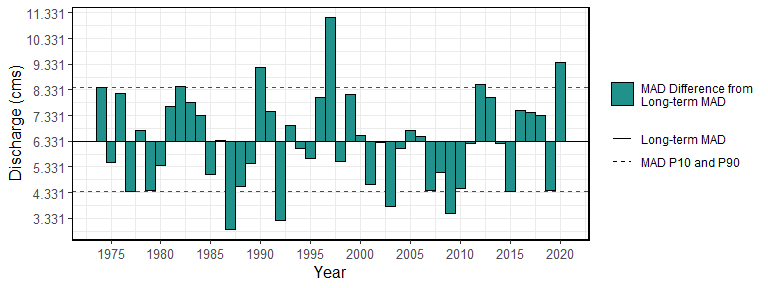## 7. Functions for Computing Analyses There are several functions that provide more in-depth analyses. These functions begin with compute_ instead of calc_ and typically produce more than just a tibble data frame of statistics, like the calc_ functions. Most of these produce a list of objects, consisting of both tibbles and plots. There are three groups of analysis functions: annual trending, annual volume frequency analyses, and a full analysis (of most fasstr functions). There is a separate vignette for each analysis type to provide more information. ### Volume Frequency Analyses There are five fasstr functions that perform various volume frequency analyses. Frequency analyses are used to determine probabilities of events of certain sizes (typically annual high or low flows). The analyses produce plots of event series and computed quantiles fitted from either Log-Pearson Type III or Weibull probability distributions. See the frequency analysis vignette for more information. The compute_annual_frequencies() performs an annual daily (or selected duration using roll_days argument) low-flow (by default) or high-flow (using use_max = TRUE argument) frequency analysis on annual series. This analysis uses the daily mean lows or highs. The compute_hydat_peak_frequencies() function performs an annual instantaneous low (by default) or high peak frequency analysis. The data argument cannot be used for the HYDAT peak analysis. Both functions output several objects in a list: 1.$Freq_Analysis_Data - Tibble of computed annual minimums (or maximums)
2. $Freq_Plot_Data - Tibble of plotting coordinates used in the frequency plot 3.$Freq_Plot - ggplot2 object of the frequency plot
4. $Freq_Fitting - List of fitdistrplus objects of the fitted distributions. 5.$Freq_Fitted_Quantiles - Tibble with fitted quantiles.

The compute_frequency_quantile() function performs annual daily (or selected duration) low-flow (by default) or high-flow (using use_max = TRUE argument) frequency analysis on annual series but only returns the fitted quantile based on the selected return period. Both the numeric arguments roll_days and return_period are required. It results in a single value. For example, supplying roll_days = 7 and return_period = 10 to the function with a data set will return the 7-day low-flow with a 10-year return period (i.e. 7Q10).

To compute a volume frequency analysis on custom data, use the compute_frequency_analysis() function. The data points to be used in the analysis must be provided in a data frame with a column of events (or years), the flow values (values), and the measure (or the type of value it is, “7-day lows”, for example. All other data filtering options are not included.

### Full Analysis

If desired, a suite of fasstr functions can be computed using the compute_full_analysis(), producing lists of tables and plots organized in lists by analysis type. write_full_analysis() will create both all the objects and also write data to your computer, in Excel-ready formats and image files. The filetypes of plots and tables can be set using the plot_filetype and table_filetype arguments, respectively. See the full analysis vignette for more information on customizing the analyses and statistics.

The plots and tables are grouped into the following analyses:

1. Screening
2. Long-term
3. Annual
4. Monthly
5. Daily
6. Annual Trends
7. Low-flow Frequencies

## 8. Customizing Functions with Arguments - Data Filtering and Options

While tidying and filtering data to desired parameters or time periods can be completed to flow data frames prior to passing them onto fasstr functions, a suite of function arguments have been provided to allow for in-function customization of tidying and filtering. Described here are some of the options available in fasstr functions on how to handle missing dates, filter for specific years or months, and select desired statistics from some of the fasstr functions. Not all functions have all these options see the documentation for each function usage (can also use ?calc_annual_stats to see documentation in R).

### Handling Missing Dates

Most functions will automatically (ignore_missing = FALSE) not calculate a statistic for a given period (a year or month or day of year, for example) if there is a date with missing data (NA value) and will result in an NA value or will not plot (base na.rm = FALSE). For example, if there at least one missing day for a given year, an annual statistic will not be calculated for that year. A warning message will appear in the console indicating as such to ensure the user is aware of missing data. See the following code for an example with missing dates:

calc_annual_stats(station_number = "08NM116")

If you want to calculate the statistics regardless of the number of missing dates per time period, use the ignore_missing = TRUE argument.

calc_annual_stats(station_number = "08NM116",
ignore_missing = TRUE)

Starting with fasstr 0.4.0, to allow a certain percentage of missing dates per period and still calculate a statistic, the argument allow_missing (and allow_missing_annual and allow_missing_monthly in come cases) will override the ignore_missing argument in certain functions. A numeric value between 0 and 100 indicating the percentage of missing dates allowed to be included is provided to the argument to calculate a statistic (0 to 100 percent). For example, if 3-4 days of missing dates are permitted per year to calculate annual means, percentiles or extremes, then 1% of days can be applied as allowed_missing = 1.

To maintain usage of ignore_missing, if ignore_missing = FALSE then it defaults to 0 (zero missing dates allowed), and if ignore_missing = TRUE then it defaults to 100 (any missing dates allowed). This argument is included only in functions that calculate annual or monthly means, percentiles, minimums, and maximums including various calc_annual_* and plot_annual_* functions, calc_monthly_stats(), plot_monthly_stats(), and most compute_* functions. See function documentation to see if included. The following example allows the data to have 25%, or ~91 days, of missing dates, to calculate annual statistics:

calc_annual_stats(station_number = "08NM116",
allowed_missing = 25)

### Dates Filtering

There are several options in the function that allow you choose year options and to filter for specific time periods. If there is a specific period, years or months, to be analyzed there are several options to customize the data supplied. While filtering of data can be done to your flow data set prior supplying it to a function (using dplyr filtering, for example), these options provide quick solutions for in-function filtering that can be incorporated into a workflow.

#### Water year and start month

By default, the functions will analyze/group/filter data by calendar years (Jan-Dec). However, some analyses require use of water years, or hydrologic years, starting in other months. If use of water years is desired not starting in January, then set water_year_start with a month other than 1. The water year is identified by the calendar year in which it ends. For example, a water year from Oct 2000 to Sep 2001 would be water year 2001.

Example of a default water year, starting in October:

calc_annual_stats(station_number = "08NM116",
ignore_missing = TRUE,
water_year_start = 9)

Example of a water year starting in August:

calc_annual_stats(station_number = "08NM116",
ignore_missing = TRUE,
water_year_start = 8)

#### Selecting and excluding years

To specify select years used in your analysis, the start_year and end_year arguments (providing a single value) can filter the years. Using the exclude_years argument (providing a single or vector of years) will allow you to remove certain years from the analysis. Leaving these arguments blank will include all years in the data set for the analysis.

Example of filtering for start and end years:

calc_annual_stats(station_number = "08NM116",
start_year = 1980,
end_year = 2010)

Examples of removing certain years (outliers, bad data, etc.) using exclude_years:

calc_annual_stats(station_number = "08NM116",
start_year = 1980,
end_year = 2010,
exclude_years = 1982)
calc_annual_stats(station_number = "08NM116",
start_year = 1980,
end_year = 2010,
exclude_years = c(1982:1984))

#### Using only years with complete data

If your data has missing dates, but you would like to use only those years with complete data, some functions utilize the complete_years argument where the data will automatically be filtered for years with complete data and statistics will be calculated. Only years with complete data will be included into the following example.

calc_longterm_daily_stats(station_number = "08NM116",
complete_years = TRUE)

Some functions, like below, require only years with complete data (statistics are based on full years of data), so years with missing dates will be automatically ignored:

calc_annual_flow_timing(station_number = "08NM116")

#### Selecting for months

Most functions allow you to specify select months used in your analysis, using the months argument. By providing a vector of months (1 through 12) only those months will be used in an analysis. For example, using the months argument with the calc_annual_stats() function will calculate the annual statistics for only those months listed. So, if summer statistics are required you supply months = 6:8 to the function. Leaving this arguments blank will include all months in the data set for the analysis. As of fasstr 0.4.0, the months argument is now included in all calc_, plot_, and compute_ functions to allow for selecting of specific months in all analyses, including calc_all_annual_stats() and compute_annual_trends().

Example of filtering for months June through August:

calc_annual_stats(station_number = "08NM116",
start_year = 1980,
end_year = 2010,
months = 6:8)

Example of flow timing / center of volume in winter/spring months:

calc_flow_timing(station_number = "08NM116",
start_year = 1980,
end_year = 2010,
months = 1:7)

A few functions, including the calc_longterm_daily_stats(), plot_longterm_daily_stats(), and plot_flow_duration() functions will allow you to add a customized time period to your data frame or plot. Using the custom_months argument you can list a vector of months (numeric 1:12). By default, the data will be labelled as “Custom-Months” but can be customized by providing a character string to the custom_months_label argument.

Example of custom months and labeling:

calc_longterm_daily_stats(station_number = "08NM116",
start_year = 1980,
end_year = 2010,
custom_months = 6:8,
custom_months_label = "Summer")

### Rolling averages

Some functions allow you to specify analyzing the data using rolling mean data as opposed to the daily means. For those functions with the roll_days and roll_align arguments, analyses will be computed on the daily mean by default (can leave them blank if so). If choosing to conduct an analysis on 7-day rolling means, you would set roll_days = 7. Some functions allow multiple rolling days to be provided (see function documentation). The roll_align argument determines the direction of the rolling mean: see the “Adding rolling means” portion in Section 4 to see how the roll_days and roll_align work together.

Example of a 7-day rolling mean analysis (single roll_days use):

calc_annual_stats(station_number = "08NM116",
start_year = 1980,
end_year = 2010,
roll_days = 7)

Example of a 7- and 30-day rolling mean analysis (multiple roll_days use):

plot_annual_lowflows(station_number = "08NM116",
start_year = 1980,
end_year = 2010,
roll_days = c(7,30))[]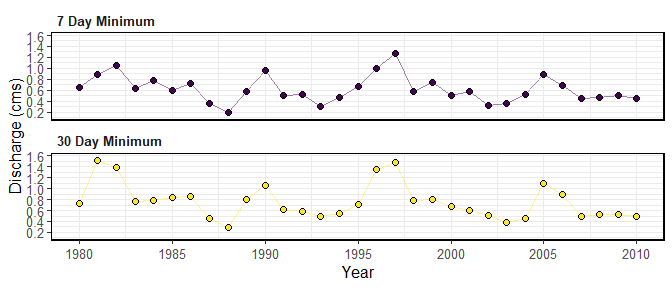### Percentiles and other statistics

Each fasstr function comes with their default statistics to be calculated. While some cannot be changed (some plotting functions), most have the ability to customize what is calculated. Look up the default settings for each function in their documentation (?calc_longterm_daily_stats for example).

By default, the basic summary statistics functions will calculate the mean, median, maximum, and minimum values for each time period; these will automatically be calculated can cannot be removed by an argument option (can remove afterwards if necessary). These functions also calculate default percentiles, which can be customized by changing the desired percentiles by providing a numeric vector of numbers (between 0 and 100) to the percentiles argument.

This example shows the default percentiles for the calc_annual_stats() function (10 and 90th percentiles):

calc_annual_stats(station_number = "08NM116",
start_year = 1980,
end_year = 2010)

This example shows custom percentiles for the calc_annual_stats() function (5 and 25th percentiles):

calc_annual_stats(station_number = "08NM116",
start_year = 1980,
end_year = 2010,
percentiles = c(5,25))

The following are some examples of how to customize results from other types of functions. See function documentations for full argument uses.

Example of calculating dates of the 10 and 20 percent of total annual flow:

calc_annual_flow_timing(station_number = "08NM116",
start_year = 1980,
end_year = 2010,
percent_total = c(10,20))

Example of plotting the number of normal and above/below normal days per year of the 10th and 90th percentiles (25th and 75th percentiles are default):

plot_annual_normal_days(station_number = "08NM116",
start_year = 1980,
end_year = 2010,
normal_percentiles = c(10,90))
$Annual_Normal_Days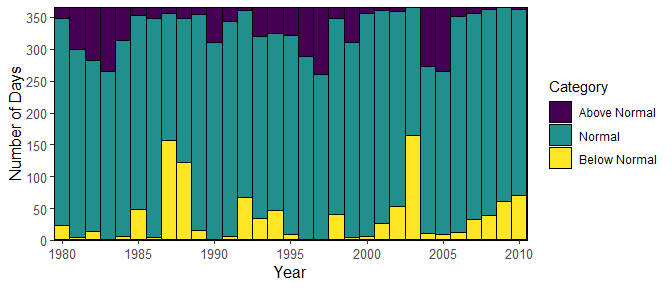#### Data frame options An option when working with the functions that produce data frames is to transpose the rows and columns of the data. Most functions by default provide data results such there are columns of statistics for each station and time period. See the example here: calc_longterm_daily_stats(station_number = "08NM116", start_year = 1980, end_year = 2010)  STATION_NUMBER Month Mean Median Maximum Minimum P10 P90 1 08NM116 Jan 1.201563 0.9650 9.50 0.160 0.5480 1.850 2 08NM116 Feb 1.146177 0.9675 4.41 0.140 0.4890 1.970 3 08NM116 Mar 1.818723 1.3800 9.86 0.380 0.7200 3.700 4 08NM116 Apr 8.333600 6.2250 37.90 0.505 1.5400 17.810 5 08NM116 May 23.585036 20.9000 74.40 3.830 9.3700 40.800 6 08NM116 Jun 21.291149 19.4000 84.50 0.450 6.0990 38.630 7 08NM116 Jul 6.421402 3.9400 54.50 0.332 1.0200 14.700 8 08NM116 Aug 2.114699 1.5700 13.30 0.427 0.7790 4.210 9 08NM116 Sep 2.206682 1.6200 14.60 0.364 0.7397 4.352 10 08NM116 Oct 2.100921 1.6500 15.20 0.267 0.8030 3.950 11 08NM116 Nov 2.024817 1.7100 11.70 0.260 0.5618 3.781 12 08NM116 Dec 1.313862 1.0800 7.30 0.342 0.5000 2.370 13 08NM116 Long-term 6.141736 1.8900 84.50 0.140 0.6850 19.300 In some circumstances, however, it may be more convenient to wrangle the data such that there are columns for stations (or groupings) and a single column with all statistics, and then the values are placed in columns for each respective time period. See the following example when setting transpose = TRUE. calc_longterm_daily_stats(station_number = "08NM116", start_year = 1980, end_year = 2010, transpose = TRUE)  STATION_NUMBER Statistic Jan Feb Mar Apr May Jun 1 08NM116 Mean 1.201563 1.146177 1.818723 8.3336 23.58504 21.29115 2 08NM116 Median 0.965000 0.967500 1.380000 6.2250 20.90000 19.40000 3 08NM116 Maximum 9.500000 4.410000 9.860000 37.9000 74.40000 84.50000 4 08NM116 Minimum 0.160000 0.140000 0.380000 0.5050 3.83000 0.45000 5 08NM116 P10 0.548000 0.489000 0.720000 1.5400 9.37000 6.09900 6 08NM116 P90 1.850000 1.970000 3.700000 17.8100 40.80000 38.63000 Jul Aug Sep Oct Nov Dec Long.term 1 6.421402 2.114699 2.206682 2.100921 2.024817 1.313862 6.141736 2 3.940000 1.570000 1.620000 1.650000 1.710000 1.080000 1.890000 3 54.500000 13.300000 14.600000 15.200000 11.700000 7.300000 84.500000 4 0.332000 0.427000 0.364000 0.267000 0.260000 0.342000 0.140000 5 1.020000 0.779000 0.739700 0.803000 0.561800 0.500000 0.685000 6 14.700000 4.210000 4.352000 3.950000 3.781000 2.370000 19.299999 #### Plotting options ##### Logarithmic discharge scale Depending on the plotting function, discharge data will be plotted using a linear or a logarithmic scale (depending on the scale of data). This can be altered using the log_discharge argument. Here is example of plotting with a linear scale (default log_discharge = FALSE): plot_annual_stats(station_number = "08NM116", start_year = 1980, end_year = 2010) $Annual_Statistics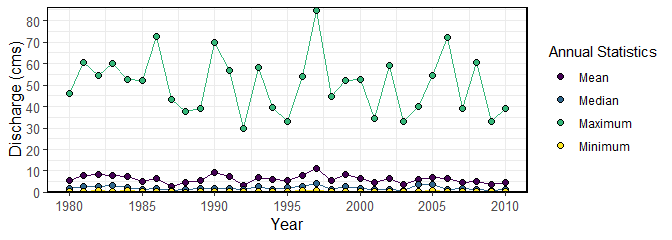Set the discharge scale to be logarithmic (log_discharge = TRUE):

plot_annual_stats(station_number = "08NM116",
start_year = 1980,
end_year = 2010,
log_discharge = TRUE)
$Annual_Statistics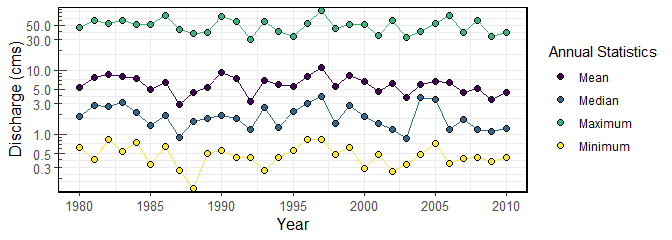##### Including a standard title on the plot The logical include_title argument adds the station number (or grouping identifier from the groupings argument), and in some cases the statistics as well. The argument’s default is FALSE. Example of including a title when plotting (include_title = TRUE): plot_annual_stats(station_number = "08NM116", start_year = 1980, end_year = 2010, include_title = TRUE) $Annual_StatisticsExample of including a title when plotting include_title = TRUE where the statistic is also displayed:

plot_monthly_stats(station_number = "08NM116",
start_year = 1980,
end_year = 2010,
include_title = TRUE)[]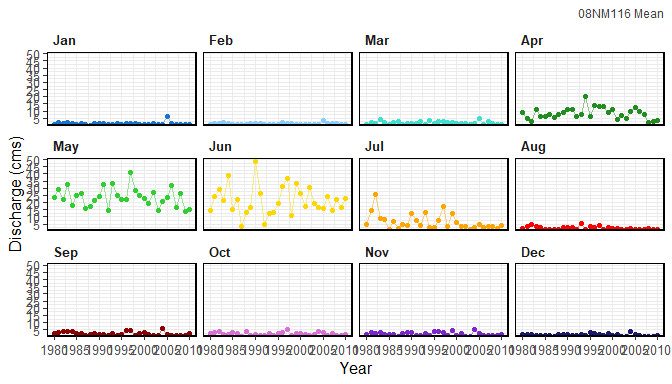Customizing a plot by using additional ggplot2 functions:

library(ggplot2)
Warning: package 'ggplot2' was built under R version 4.2.3
# Create the plot list and extract the plot using []
plot <- plot_daily_stats(station_number = "08NM116", start_year = 1980)[]

# Customize the plot with various ggplot2 functions
plot +
geom_hline(yintercept = 1.5, colour = "red", linetype = 2, size = 1) +
geom_vline(xintercept = as.Date("1900-03-01"), colour = "darkgray", linetype = 1, size = 0.5) +
geom_vline(xintercept = as.Date("1900-08-05"), colour = "darkgray", linetype = 1, size = 0.5) +
ggtitle("Mission Creek Annual Hydrograph") +
ylab("Flow (cms)")
Warning: Using size aesthetic for lines was deprecated in ggplot2 3.4.0.
ℹ Please use linewidth instead.
This warning is displayed once every 8 hours.
Call lifecycle::last_lifecycle_warnings() to see where this warning was
generated.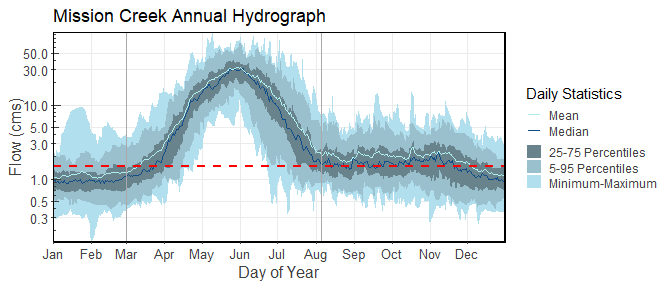## 9. Writing Tables and Plots

To support saving the fasstr tables and plots to a directory, there are several functions included in this package. These include the following:

• write_flow_data() - write a streamflow data set as a .xlsx, .xls, or .csv file
• write_results() - write a data frame as a .xlsx, .xls, or .csv file
• write_plots() - write plots from a list into a directory or PDF document
• write_objects_list() - write all tables and plots contained in a list

#### Writing a flow data set

To directly save a streamflow data set from HYDAT or your own custom data frame onto your computer, you can use the write_flow_data() function. By listing the station_number or data data frame, the data set will save a file into the working directory, unless otherwise specified using the file_name argument. If using the station_number argument and listing only one station without listing a name with file_name, the name will include the number and followed by “_daily_data.xlsx”; and if multiple stations are listed the name will be “HYDAT_daily_data.xlsx”. When using the data argument without listing a name with file_name the default name will be fasstr_daily_data.xlsx. To use another file type than “xlsx” (options are “xlsx”, “xls”, or “csv”) provide a file name using the file_name argument with the desired extension. Other argument options for this function include:

• selecting for the start and end years or dates
• choosing to use water years when selecting specific years
• selecting whether or not to fill dates with missing data with NA’s (logical fill_missing argument)
• selecting the number of digits to round the flow values (numeric digits argument)

The following will write an “xlsx” file called “08NM116_data_data.xlsx” into your working directory that includes all daily flow data from that station in HYDAT:

write_flow_data(station_number = "08NM116")

The following is an example of possible customization:

write_flow_data(station_number = "08NM116",
start_year = 1960,
end_year = 1970
fill_missing = TRUE,
file_name = "mission_creek.csv")

#### Writing a data frame

While you can use the base R write_csv() or writexl package functions to save your data, the package provides a function with options to choose for file type and the rounding of digits. To directly save a data frame onto your computer you can use the write_results() function. This function allows you to decide on file extensions of “xlsx”, “xls”, or “csv” by including it in the file_name argument when you name the file. This function also allows you to round all numeric columns by selecting the number of digits using the numeric digits argument.

annual_data <- calc_annual_stats(station_number = "08NM116")

write_results(data = annual_data,
digits = 3,
file_name = "mission_creek_annual_flows.xlsx")

#### Writing a list of plots

As all plots produced with this package are contained within lists, a function is provided to assist in saving a list of plots into either a folder, where all plot files are named by the object names within the list, or combined PDF document, using the write_plots() function. The name of the folder or PDF document is provided using the folder_name argument. If the folder does not exist, one will be created. Options to customize output size with width, height, units and dpi arguments, as similar to those in ggplots2:ggsave(), can also be used.

The following will save each annual plot as a “png” file in a folder called “Annual Plots” in the working directory:

annual_plots <- plot_annual_stats(station_number = c("08NM116","08NM242"))

write_plots(plots = annual_data,
folder_name = "Annual Plots",
plot_filetype = "png")

The following will save all annual plots as combined “pdf” document called “Annual Plots” in the working directory with each plot on a different page:

annual_plots <- plot_annual_stats(station_number = c("08NM116","08NM242"))

write_plots(plots = annual_data,
folder_name = "Annual Plots",
combined_pdf = TRUE)

If you would prefer to save the plots using other functions, like the ggplot2::ggsave() function, the desired plot must subsetted from the list first so the object provided the function is a plot object and not a list. Individual plots can be subsetted from their lists using either the dollar sign, $(e.g. one_plot <- plots$plotname), or double square brackets, [ ] (e.g. one_plot <- plots[[plotname]] or one_plot <- plots[]).

#### Writing a list of data frames and plots

As some objects produced with this package, mainly with the compute_* functions, contain lists of both data frames and ggplot2 objects, a function is provided, called write_objects_list(), to assist in saving all objects within the list into a designated directory folder, where all table and plot files are named by the object names. The name of the folder is provided using the folder_name argument. If the folder does not exist, one will be created. The file type for tables and plots are chosen using the table_filetype and plot_filetype arguments respectively. There are also options to customize plot output size with width, height, units and dpi arguments, as similar to those in ggplots2:ggsave() can also be used.

The following will save all plots and tables in a folder called “Frequency Analysis” in the working directory:

freq_analysis <- compute_annual_frequencies(station_number = "08NM116")

write_objects_list(list = freq_analysis,
folder_name = "Frequency Analysis",
plot_filetype = "png",
table_filetype = "xlsx")`